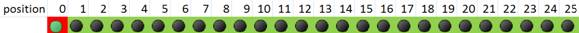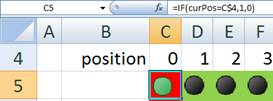## How Multi Agent Simulation Works?

There is no actual program (in term of VB code or macro) in the spreadsheet that you have downloaded, actually. All are done using pure spreadsheet with Iteration options is set as ON. In this section, I will explain the principle on how the race of multi agent work and then I will describe how to do the 'programming' of the multi agents in spreadsheet in the next section.

First I will describe the agent's properties and then I will describe the simulation or animation itself.

Each agent only has three properties: decision to move (binary), current position (0 to 25) and current clock.

Decision to move is simply random decision either to move or to stop at current simulation clock. To make the probability to move as 50%, I use the following formula:

=IF(RAND()<0.5,1,0)

Current position is the heart of the multi agent 'program'. In principle, the agent should move to the next position if the race is start and if the agent is decided to move and the current position is not the last position. Otherwise, the current position remains unchanged. If the race has not started yet, the agent position must be zero. The formulation of this principle in MS Excel is as follow:

=IF(Control="",

IF(AND(isMove=1,curPos<25),

curPos+1,

curPos),

0)

To know whether the agent is winning or not, we need to trace the current clock until the agent reach the final position or finishing line. If the last position is still zero (not filled by the agent yet) then we keep the simulation clock, otherwise we keep the last current clock. The formulation of the current clock for each agent is therefore like this:

=IF(lastPos=0,simClock,curClock)

Having the agents, now we need only two more things to do: to make the simulation clock and the animation of the race.

The race of our agents starts when the user deletes the content of the Control cell (cell C1 in the spreadsheet example). When the Control cell is filled with any character or number, the race is over and restarted. A simulation clock is simply a counter that count how many times the user press recalculation button (function key F9). This will work only if you set the options of iteration as enable and maximum iteration is set as 1 (if you are not sure how to do that, read next section of this tutorial). In the beginning or when there is no race of our multi agents, we set the simulation clock to be zero. Thus, the formula of simulation clock is

=IF(Control="",simClock+1,0)

To animate the race, we make a race field, consist of 26 cell positions. On the top of the race field, we mark the position number 0 to 26.To let the user view the animation, we put value 1 on the race field if the current position is equal to the number value of the position, otherwise the race field contain zero. As each agent have only one current position, then only one cell in the race field will contain 1 (of course, that cell must be on the lane of the agent). The formulation for the race field is shown in the figure below.To see the detail of the programming steps, read on next section of this tutorial.